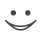# This flashcard is just one of a free flashcard set. See all flashcards!

233
13.When a stock price, S,  follows geometric Brownian motion with mean return m and volatility s what is the process follows by X where X = ln S.
A.dX = m dt + s dz
B.dX = (m−r) dt + s dz
C.dX = (m−s2) dt + s dz
D.dX = (m − s2/2) dt + s dz